# F.1 Electron-Electron Interaction

By taking the functional derivative of (F.4) with respect to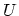one obtains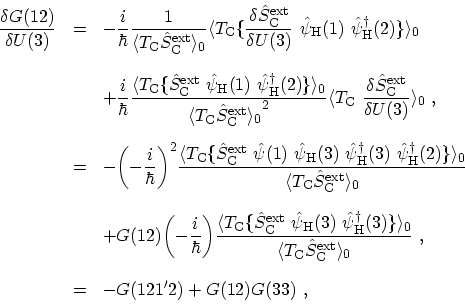(F.5)

Equation (F.5) relates the two-particle GREEN's function to the functional derivative of the single-particle GREEN's function, which allows one to write the equation of motion (F.3) as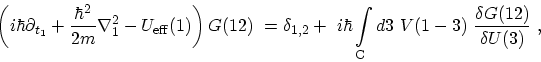(F.6)

where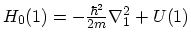and the effective potential is given by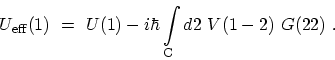(F.7)

Since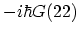is nothing but the electron density, the second term in (F.7) can be easily identified as the HARTREE potential. Exchange and correlation effects are described by the functional derivative contribution, which still requires the calculation of a two-particle GREEN's function. In order to decouple the hierarchy formally, one can introduce the single-particle self-energy. This is accomplished by the identity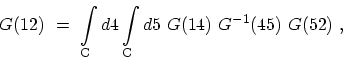(F.8)

Differentiating with respect to, one obtains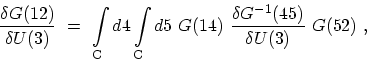(F.9)

where the following relation was used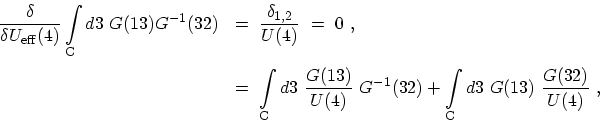(F.10)

which allows us to express the functional derivative of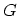by means of the functional derivative of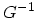. Therefore, the equations of motion can be cast into a closed form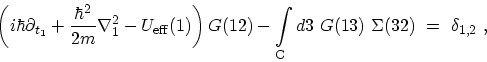(F.11)

where the self-energy is defined as(F.12)

Subsections M. Pourfath: Numerical Study of Quantum Transport in Carbon Nanotube-Based Transistors